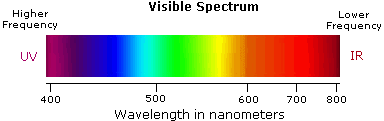# Question #afab2

Jul 16, 2014

Violet light has the shortest wavelength.

#### Explanation:

Visible light has a wavelength range of about 400 nm to 750 nm.The visible colours from shortest to longest wavelength are: violet, indigo, blue, cyan, green, yellow, orange, and red.

The spectrum is continuous and the colours merge into each other, so the cut-off ranges of the colours are somewhat arbitrary.

$\textcolor{w h i t e}{l l} \boldsymbol{\text{λ/nm"color(white)(mll)"Colour}}$
$400 - 425 \textcolor{w h i t e}{m} \text{Violet}$
$425 - 450 \textcolor{w h i t e}{m} \text{Indigo}$
$450 - 475 \textcolor{w h i t e}{m} \text{Blue}$
$475 - 495 \textcolor{w h i t e}{m} \text{Cyan}$
$495 - 560 \textcolor{w h i t e}{m} \text{Green}$
$550 - 580 \textcolor{w h i t e}{m} \text{Yellow}$
$580 - 600 \textcolor{w h i t e}{m} \text{Orange}$
$600 - 750 \textcolor{w h i t e}{m} \text{Red}$